# 13.1 Sequences and their notations  (Page 7/15)

 Page 7 / 15

$\left(\frac{12}{6}\right)!$

$\frac{12!}{6!}$

$665,280$

$\frac{100!}{99!}$

For the following exercises, write the first four terms of the sequence.

${a}_{n}=\frac{n!}{{n}^{\text{2}}}$

First four terms: $1,\frac{1}{2},\frac{2}{3},\frac{3}{2}$

${a}_{n}=\frac{3\cdot n!}{4\cdot n!}$

${a}_{n}=\frac{n!}{{n}^{2}-n-1}$

First four terms: $-1,2,\frac{6}{5},\frac{24}{11}$

${a}_{n}=\frac{100\cdot n}{n\left(n-1\right)!}$

## Graphical

For the following exercises, graph the first five terms of the indicated sequence

${a}_{n}=\frac{{\left(-1\right)}^{n}}{n}+n$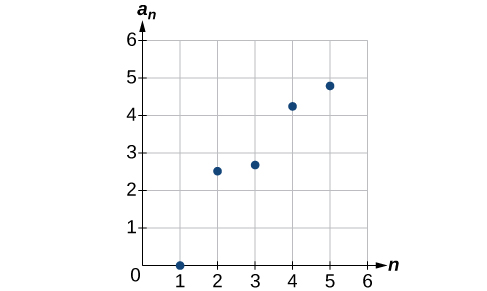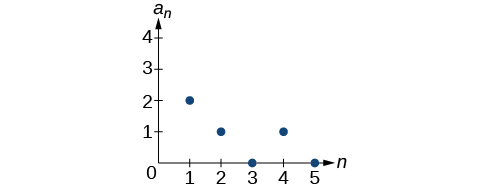${a}_{n}=\frac{\left(n+1\right)!}{\left(n-1\right)!}$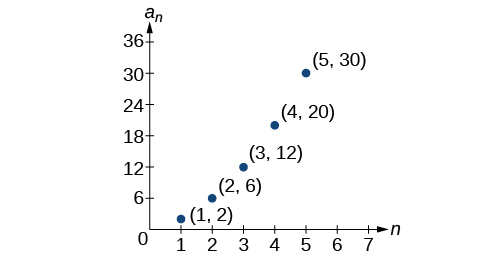For the following exercises, write an explicit formula for the sequence using the first five points shown on the graph.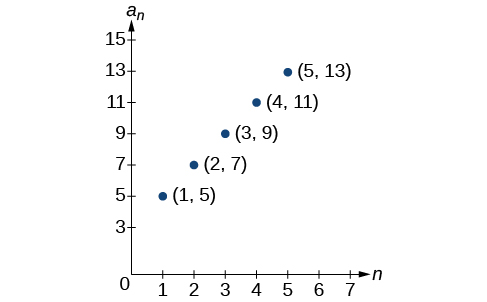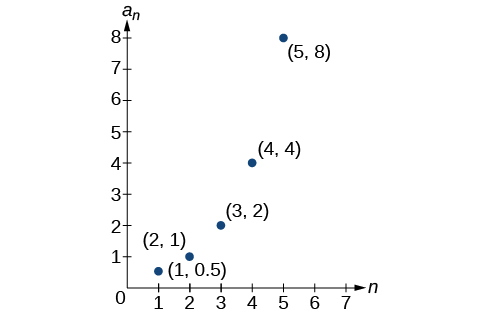${a}_{n}={2}^{n-2}$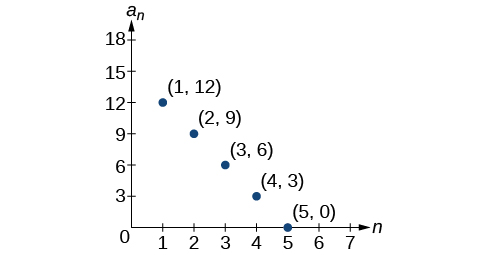For the following exercises, write a recursive formula for the sequence using the first five points shown on the graph.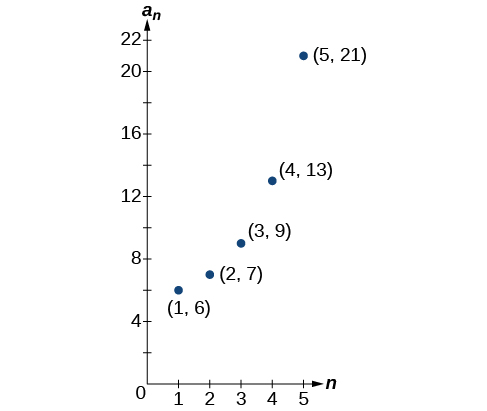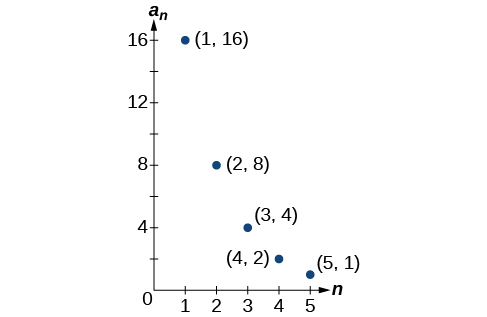## Technology

Follow these steps to evaluate a sequence defined recursively using a graphing calculator:

• On the home screen, key in the value for the initial term $\text{\hspace{0.17em}}{a}_{1}\text{\hspace{0.17em}}$ and press [ENTER] .
• Enter the recursive formula by keying in all numerical values given in the formula, along with the key strokes [2ND] ANS for the previous term $\text{\hspace{0.17em}}{a}_{n-1}.\text{\hspace{0.17em}}$ Press [ENTER] .
• Continue pressing [ENTER] to calculate the values for each successive term.

For the following exercises, use the steps above to find the indicated term or terms for the sequence.

Find the first five terms of the sequence Use the> Frac feature to give fractional results.

First five terms: $\frac{29}{37},\frac{152}{111},\frac{716}{333},\frac{3188}{999},\frac{13724}{2997}$

Find the 15 th term of the sequence

Find the first five terms of the sequence

First five terms: $2,3,5,17,65537$

Find the first ten terms of the sequence

Find the tenth term of the sequence

${a}_{10}=7,257,600$

Follow these steps to evaluate a finite sequence defined by an explicit formula. Using a TI-84, do the following.

• In the home screen, press [2ND] LIST .
• Scroll over to OPS and choose “seq(” from the dropdown list. Press [ENTER] .
• In the line headed “Expr:” type in the explicit formula, using the $\text{\hspace{0.17em}}\left[\text{X,T},\theta ,n\right]\text{\hspace{0.17em}}$ button for $\text{\hspace{0.17em}}n$
• In the line headed “Variable:” type in the variable used on the previous step.
• In the line headed “start:” key in the value of $\text{\hspace{0.17em}}n\text{\hspace{0.17em}}$ that begins the sequence.
• In the line headed “end:” key in the value of $\text{\hspace{0.17em}}n\text{\hspace{0.17em}}$ that ends the sequence.
• Press [ENTER] 3 times to return to the home screen. You will see the sequence syntax on the screen. Press [ENTER] to see the list of terms for the finite sequence defined. Use the right arrow key to scroll through the list of terms.

Using a TI-83, do the following.

• In the home screen, press [2ND] LIST .
• Scroll over to OPS and choose “seq(” from the dropdown list. Press [ENTER] .
• Enter the items in the order “Expr” , “Variable” , “start” , “end” separated by commas. See the instructions above for the description of each item.
• Press [ENTER] to see the list of terms for the finite sequence defined. Use the right arrow key to scroll through the list of terms.

For the following exercises, use the steps above to find the indicated terms for the sequence. Round to the nearest thousandth when necessary.

List the first five terms of the sequence ${a}_{n}=-\frac{28}{9}n+\frac{5}{3}.$

List the first six terms of the sequence

First six terms: $0.042,0.146,0.875,2.385,4.708$

List the first five terms of the sequence ${a}_{n}=\frac{15n\cdot {\left(-2\right)}^{n-1}}{47}$

List the first four terms of the sequence ${a}_{n}={5.7}^{n}+0.275\left(n-1\right)!$

First four terms: $5.975,32.765,185.743,1057.25,6023.521$

List the first six terms of the sequence ${a}_{n}=\frac{n!}{n}.$

## Extensions

Consider the sequence defined by ${a}_{n}=-6-8n.$ Is ${a}_{n}=-421$ a term in the sequence? Verify the result.

If $\text{\hspace{0.17em}}{a}_{n}=-421\text{\hspace{0.17em}}$ is a term in the sequence, then solving the equation $-421=-6-8n$ for $n$ will yield a non-negative integer. However, if $\text{\hspace{0.17em}}-421=-6-8n,\text{\hspace{0.17em}}$ then $n=51.875$ so ${a}_{n}=-421$ is not a term in the sequence.

What term in the sequence ${a}_{n}=\frac{{n}^{2}+4n+4}{2\left(n+2\right)}$ has the value $41?$ Verify the result.

Find a recursive formula for the sequence ( Hint : find a pattern for $\text{\hspace{0.17em}}{a}_{n}\text{\hspace{0.17em}}$ based on the first two terms.)

${a}_{1}=1,{a}_{2}=0,{a}_{n}={a}_{n-1}-{a}_{n-2}$

Calculate the first eight terms of the sequences ${a}_{n}=\frac{\left(n+2\right)!}{\left(n-1\right)!}$ and ${b}_{n}={n}^{3}+3{n}^{2}+2n,$ and then make a conjecture about the relationship between these two sequences.

Prove the conjecture made in the preceding exercise.

$\frac{\left(n+2\right)!}{\left(n-1\right)!}=\frac{\left(n+2\right)·\left(n+1\right)·\left(n\right)·\left(n-1\right)·...·3·2·1}{\left(n-1\right)·...·3·2·1}=n\left(n+1\right)\left(n+2\right)={n}^{3}+3{n}^{2}+2n$

Find the possible value of 8.5 using moivre's theorem
which of these functions is not uniformly cintinuous on (0, 1)? sinx
which of these functions is not uniformly continuous on 0,1
solve this equation by completing the square 3x-4x-7=0
X=7
Muustapha
=7
mantu
x=7
mantu
3x-4x-7=0 -x=7 x=-7
Kr
x=-7
mantu
9x-16x-49=0 -7x=49 -x=7 x=7
mantu
what's the formula
Modress
-x=7
Modress
new member
siame
what is trigonometry
deals with circles, angles, and triangles. Usually in the form of Soh cah toa or sine, cosine, and tangent
Thomas
solve for me this equational y=2-x
what are you solving for
Alex
solve x
Rubben
you would move everything to the other side leaving x by itself. subtract 2 and divide -1.
Nikki
then I got x=-2
Rubben
it will b -y+2=x
Alex
goodness. I'm sorry. I will let Alex take the wheel.
Nikki
ouky thanks braa
Rubben
I think he drive me safe
Rubben
how to get 8 trigonometric function of tanA=0.5, given SinA=5/13? Can you help me?m
More example of algebra and trigo
What is Indices
If one side only of a triangle is given is it possible to solve for the unkown two sides?
cool
Rubben
kya
Khushnama
please I need help in maths
Okey tell me, what's your problem is?
Navin
the least possible degree ?
(1+cosA)(1-cosA)=sin^2A
good
Neha
why I'm sending you solved question
Mirza
Teach me abt the echelon method
Khamis
exact value of cos(π/3-π/4)
What is differentiation?ByByByBy